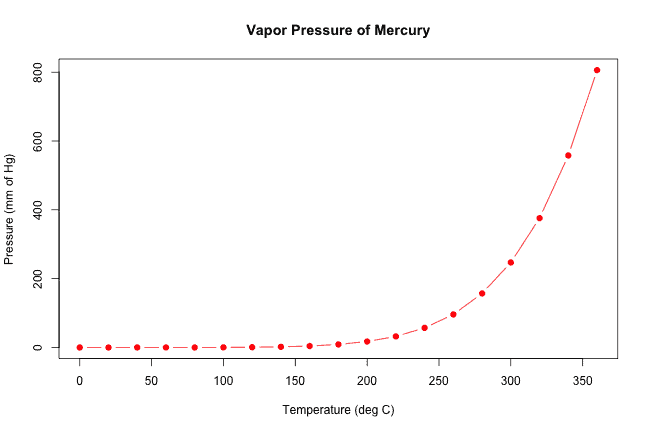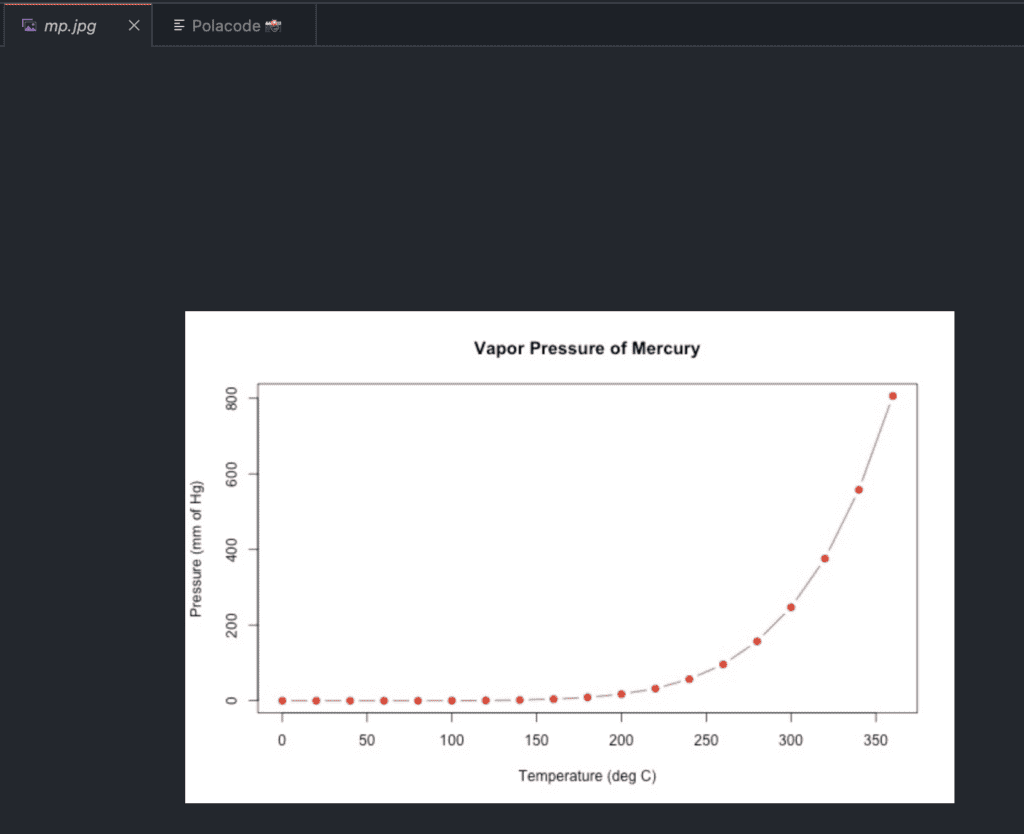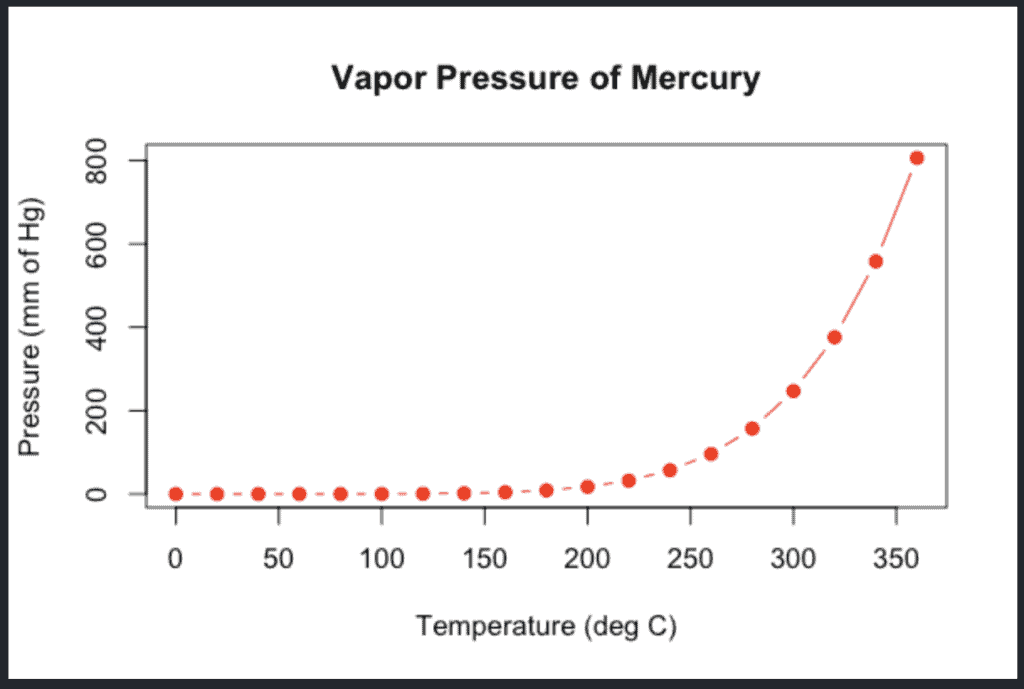# How to Save a Plot in R

To save a plot in R, follow these steps.

1. Call a function to open a new graphics file, such as png(), jpg(), bmp(), or tiff().
2. In the next step, call the plot() function to generate the graphics image.
3. At last, call the dev.off() function to close the graphics file.

If you don’t provide an external path, it will save in your current directory.

``````png(filename = "mp.png", width = 625, height = 400)

plot(pressure, col = "red", pch = 19, type = "b",
main = "Vapor Pressure of Mercury",
xlab = "Temperature (deg C)",
ylab = "Pressure (mm of Hg)")

dev.off()``````

Output## Save as a Jpeg image

To save a plot as a jpeg image, you can use the “jpeg()” function.

``````jpeg(filename = "mp.jpg", width = 625, height = 400)

plot(pressure,
col = "red", pch = 19, type = "b",
main = "Vapor Pressure of Mercury",
xlab = "Temperature (deg C)",
ylab = "Pressure (mm of Hg)"
)

dev.off()``````

Output## Save as bmp image

We can specify the size of our image in inch, cm, or mm with the argument units and specify ppi with res.

``````bmp(
file = "plot3.bmp",
width = 6, height = 4, units = "in", res = 100
)

plot(pressure,
col = "red", pch = 19, type = "b",
main = "Vapor Pressure of Mercury",
xlab = "Temperature (deg C)",
ylab = "Pressure (mm of Hg)"
)

dev.off()``````

Output## Save as tiff image

To save in the tiff format, we only need to change the first line to tiff(filename = “plot3”).

``````tiff(
file = "plot3.tiff",
width = 6, height = 4, units = "in", res = 100
)

plot(pressure,
col = "red", pch = 19, type = "b",
main = "Vapor Pressure of Mercury",
xlab = "Temperature (deg C)",
ylab = "Pressure (mm of Hg)"
)

dev.off()``````

That’s it.